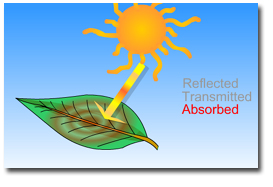# Leaf Optical - Definition

Light can be reflected, transmitted or absorbed by a canopy element.

Considering percentages:

 r + t + a = 1 
where
r =  reflected energy
t = transmitted energy
a = absorbed energy
Absorbed energy is not easy to measure so, it is calculated base on
 a = 1 - r + t [1']

Measurement technique of optical properties of leaves or canopy elements with an integrating sphere depends on three conditions:

• Is the sample translucent enough so light can transmit through the sample?
• Is there significant difference in the optical properties of the different sides of the sample (top vs. bottom; adaxial vs. abaxial)?
• Do the leaf, leaves or canopy element(s) completely encompass the beam of light from the light source?

For the first condition, if the sample IS translucent enough for light to transmit through the sample then transmitted, reflected and reference measurements can be taken. If the sample is not translucent then only reflected and reference measurements need to be taken. Transmitted energy is assumed zero.

For the second condition, if there is significant differences in the optical properties of the surfaces of the sample then all side of the sample should be measured.  Multiple  reference measurements should also be measured to represent the effect of each sides contribution to the internal reflectance of the integrating sphere.

For the third condition, a sample can fall into three categories:

1. Sample completely covers the light source's beam
2. Sample is narrower than the light source's beam, but is long enough to reach across the sample port of the integrating sphere.
3. Sample is narrower than the light source's beam and shorter than the integrating sphere's sample port.

Daughtry et al. (1989) and Mesarch et al. (1999) describe measurement techniques and revised measurement techniques for measurements of these three categories.